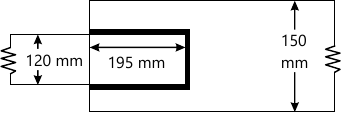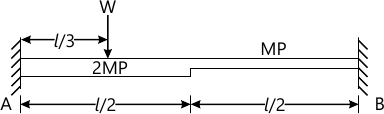MORE IN Design of Steel Structures
VTU Civil Engineering (Semester 7)
Design of Steel Structures
June 2015
Total marks: --
Total time: --
INSTRUCTIONS
(1) Assume appropriate data and state your reasons
(2) Marks are given to the right of every question
(3) Draw neat diagrams wherever necessary

1 (a) Briefly explain the failure criteria for steel.
5 M
1 (b) mention the advantages (any five) and disadvantages (any three) of steel members.
5 M
1 (c) Explain briefly:
i) Partial safety factors for loads.
ii) characteristic strength of steel.
iii) Plastic, compact and semi-compact members.
10 M

4 M
2 (b) Two plates of 16mm thickness have been connected in a lap joint using HSFG bolts. Design the joint so as to transmit a pull equal to the full strength of plate. Adopt 16mm diameter bolts. Assume edge distance of 30mm, pitch=60mm.
8 M
2 (c) Determine nominal shear capacity, design shear strength, nominal bearing strength and design strength in bearing for M16, property class 8.8 bolts assuming bolt threads outside the shear plane. Bolt are connected to 12mm thick plates. Assume end distance of bolt = 300 mm, pitch = 80 mm, fo=410 Mpa, Asb= 201 mm2.
8 M

3 (a) Determine size of weld, pull transmitted length of weld and tensile strength of plate (smaller width) for the plates shown in Fig. Q3(a) if the plates are of 10mm thick each. Assume suitable partial safety factors ad yield stress for weld and steel plates.10 M
3 (b) Determine the suitable weld size for the connection shown in Fig. Q3(b).
:IMAGE-
10 M

4 (a) Define plastic hinge, collapse load, shape factor, a mechanism and plastic moment.
5 M
4 (b) State lower and upper bound theorem.
5 M
4 (c) Determine:
i) Number of possible plastic hinges
ii) Number of independent mechanism and
iii) Collapse load for the beam
Shown in Fig. Q4 (c)10 M

5 (a) Mention the steps followed for designing a Tie member.
5 M
5 (b) Design a single angle to carry a tension of 100kN. Adopt M20, Grade 'C', property class 4.6 bolts. Assume yield and ultimate strengths of the steel as 250MPa and 410MPa respectively. Also, check for rupture and shear. Assume Asb for M20 holt=314 mm2, pitch = 60mm, edge distance = 40mm.
15 M

6 (a) Determine the design load carrying capacity of a single angle (discontinuous) ISA 50×50×5 used as compression member in a roof truss connected to a 10mm gusset by two bolts. The center to center distance between and connections is 1.50 mt. Assume fy=250 Mpa.
10 M
6 (b) Determine the load carrying capacity of two angles ISA 70×70×6mm connected to both sides of gusset using two bolts. Sketch the details. Adopt 8mm gusset plate. Length of the angle be 2.50mt.
10 M

7 (a) Explain briefly steps involved while designing a slab base and a Gausseted base. Also, sketch the details (without scale).
10 M
7 (b) Determine area of slab base. Dimensions of the slab and thickness of the base plate for a steel column carrying factored axial load (compression) of 1000kN. Pedestal is of concrete M20. Adopt fy=250 Mpa for steel.
10 M

8 (a) What is web bulking and web crippling? Explain briefly with sketches.
5 M
8 (b) Briefly explain the steps involved in designing a steel beams as per IS800-2007.
5 M
8 (c) Determine the design bending strength of ISMB [email protected] 61.6 kg/m having effective span 10m. Is the section is plastic or compact?
10 M

More question papers from Design of Steel Structures